# UNITV Logic Programming Languages Functional Programming Languages By

• Slides: 43UNIT-V Logic Programming Languages & Functional Programming Languages By Mr. T. M. Jaya Krishna M. Tech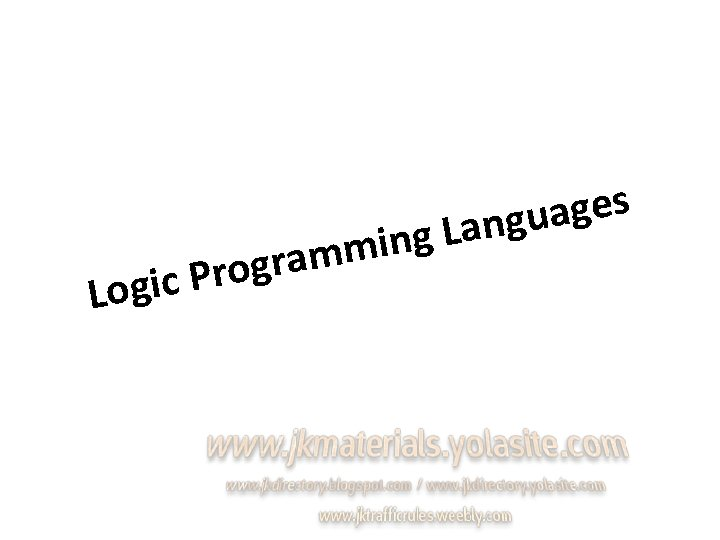Lo g n i m m a r g o r P c i g s e g a u g n a LPredicate Calculus • Proposition – logical stmt • may | may true – Objects & relationships among objects (consists) • Formal logic – Developed provide method describing propositions, w goal = allowing • formally stated propositions checked validity • Symbolic logic – used 3 basic needs = formal logic to: • express propositions • express the relationships b/n propositions • describe h? new propositions c inferred other propositions that r assumed true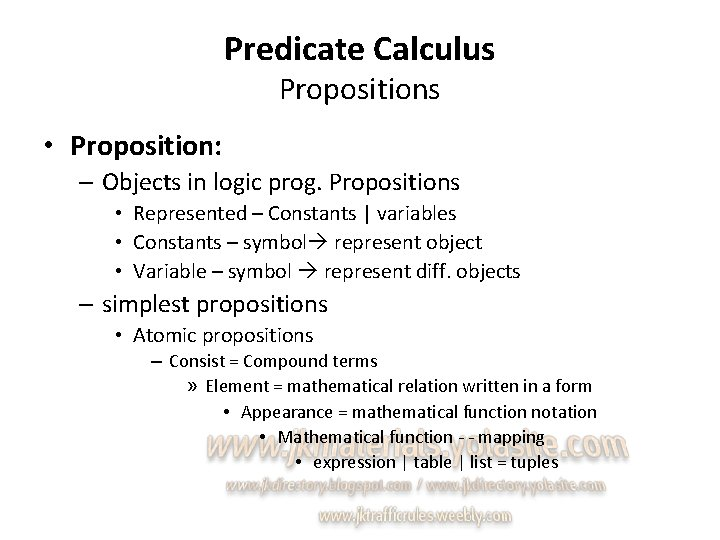Predicate Calculus Propositions • Proposition: – Objects in logic prog. Propositions • Represented – Constants | variables • Constants – symbol represent object • Variable – symbol represent diff. objects – simplest propositions • Atomic propositions – Consist = Compound terms » Element = mathematical relation written in a form • Appearance = mathematical function notation • Mathematical function - - mapping • expression | table | list = tuplesPredicate Calculus • Propositions stated in 2 modes – Defined true – Truth = proposition - - something i. e. determined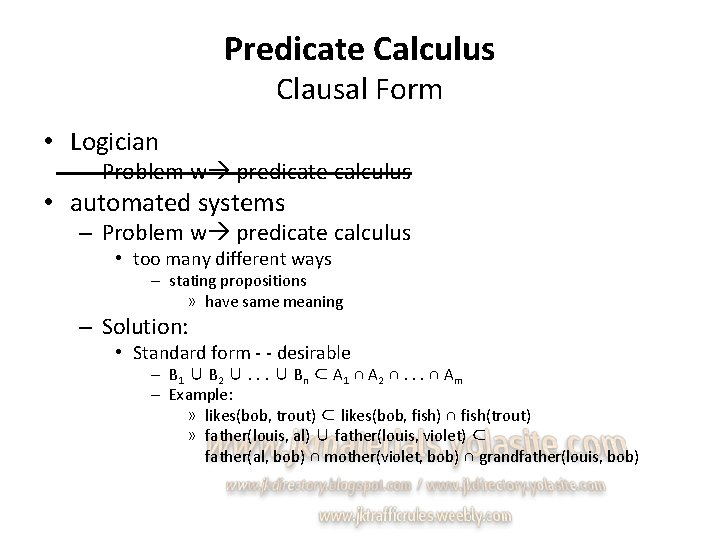Predicate Calculus Clausal Form • Logician – Problem w predicate calculus • automated systems – Problem w predicate calculus • too many different ways – stating propositions » have same meaning – Solution: • Standard form - - desirable – B 1 ∪ B 2 ∪. . . ∪ B n ⊂ A 1 ∩ A 2 ∩. . . ∩ Am – Example: » likes(bob, trout) ⊂ likes(bob, fish) ∩ fish(trout) » father(louis, al) ∪ father(louis, violet) ⊂ father(al, bob) ∩ mother(violet, bob) ∩ grandfather(louis, bob)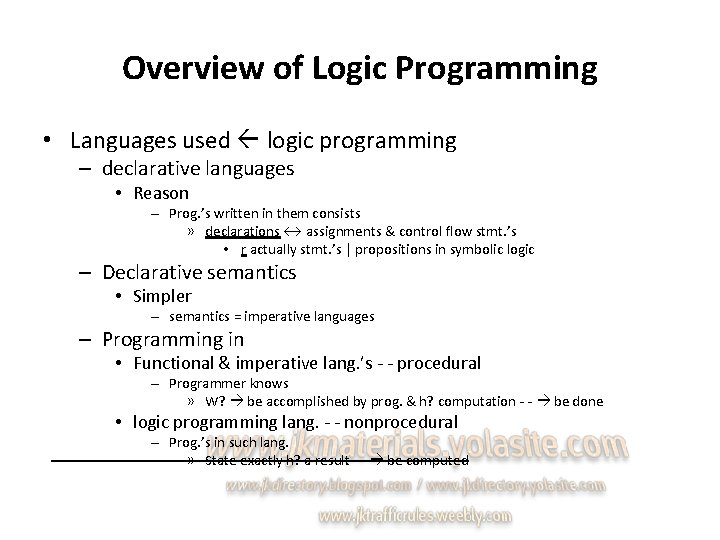Overview of Logic Programming • Languages used logic programming – declarative languages • Reason – Prog. ’s written in them consists » declarations ↔ assignments & control flow stmt. ’s • r actually stmt. ’s | propositions in symbolic logic – Declarative semantics • Simpler – semantics = imperative languages – Programming in • Functional & imperative lang. ’s - - procedural – Programmer knows » W? be accomplished by prog. & h? computation - - be done • logic programming lang. - - nonprocedural – Prog. ’s in such lang. » State exactly h? a result - - be computedThe Origins of Prolog • Alain Colmerauer & Phillippe Roussel – At University = Aix-Marseille • Assistance Robert Kowalski – At University = Edinburgh » Developed fundamental design = prolog – Colmerauer & Roussel • natural lang. processing – Kowalski • Automated theorem proving – Collaboration b/n Aix-Marseille & Edinburgh • Continued – mid-1970’s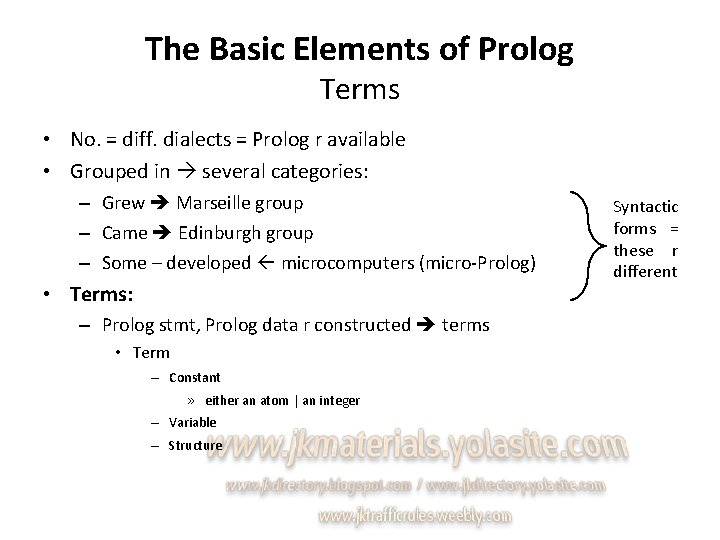The Basic Elements of Prolog Terms • No. = diff. dialects = Prolog r available • Grouped in several categories: – Grew Marseille group – Came Edinburgh group – Some – developed microcomputers (micro-Prolog) • Terms: – Prolog stmt, Prolog data r constructed terms • Term – Constant » either an atom | an integer – Variable – Structure Syntactic forms = these r different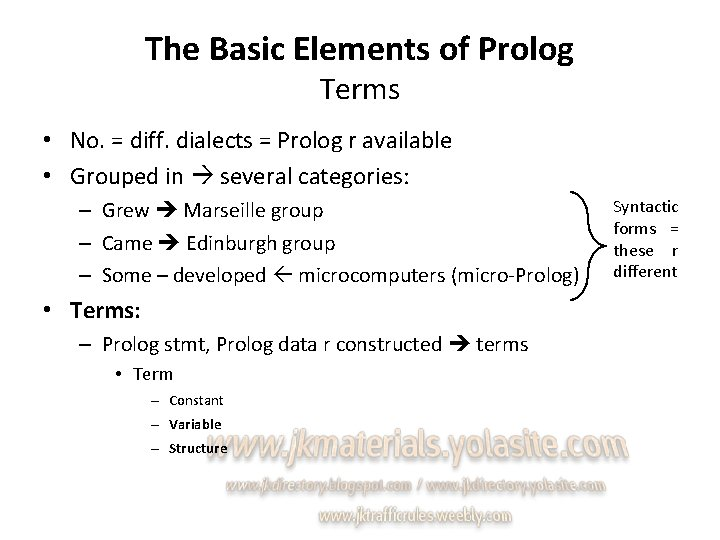The Basic Elements of Prolog Terms • No. = diff. dialects = Prolog r available • Grouped in several categories: – Grew Marseille group – Came Edinburgh group – Some – developed microcomputers (micro-Prolog) • Terms: – Prolog stmt, Prolog data r constructed terms • Term – Constant – Variable – Structure Syntactic forms = these r different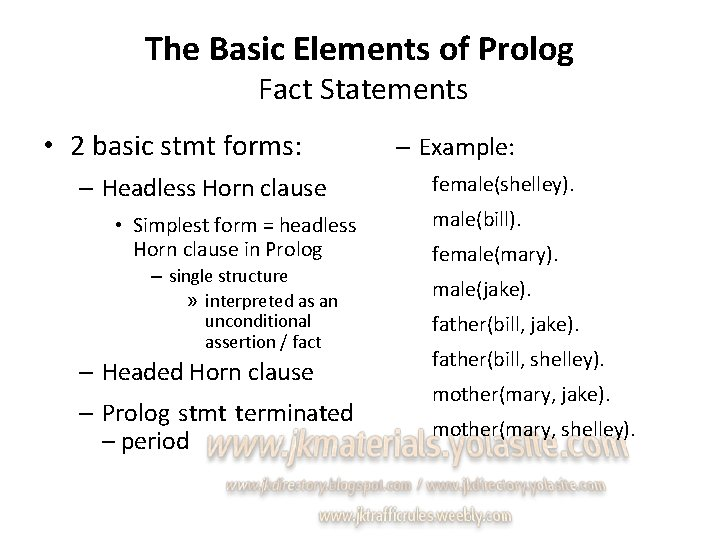The Basic Elements of Prolog Fact Statements • 2 basic stmt forms: – Headless Horn clause • Simplest form = headless Horn clause in Prolog – single structure » interpreted as an unconditional assertion / fact – Headed Horn clause – Prolog stmt terminated – period – Example: female(shelley). male(bill). female(mary). male(jake). father(bill, shelley). mother(mary, jake). mother(mary, shelley).The Basic Elements of Prolog Rule Statements • Other basic form = prolog stmt – Constructing database corresponds headed Horn clause – This form c related known theorem in mathematics • Conclusion c drawn – Set = given conditions satisfied – Right side - - antecedent (if part) – Left side - - consequent (then part) – general form (Prolog headed Horn clause stmt): consequence : - antecedent_expression. – Example: ancestor(mary, shelley) : - mother(mary, shelley).The Basic Elements of Prolog Rule Statements • Other basic form = prolog stmt – Constructing database corresponds headed Horn clause – This form c related known theorem in mathematics • Conclusion c drawn – Set = given conditions satisfied – Right side - - antecedent (if part) – Left side - - consequent (then part) – general form (Prolog headed Horn clause stmt): consequence : - antecedent_expression. – Example: ancestor(mary, shelley) : - mother(mary, shelley).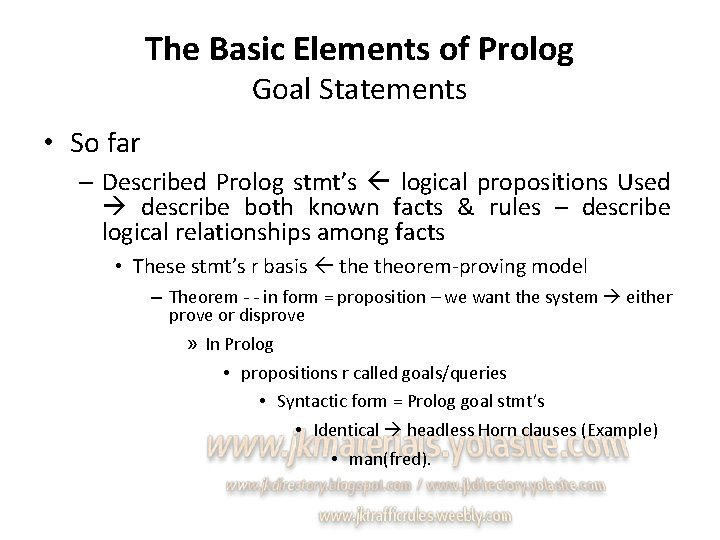The Basic Elements of Prolog Goal Statements • So far – Described Prolog stmt’s logical propositions Used describe both known facts & rules – describe logical relationships among facts • These stmt’s r basis theorem-proving model – Theorem - - in form = proposition – we want the system either prove or disprove » In Prolog • propositions r called goals/queries • Syntactic form = Prolog goal stmt’s • Identical headless Horn clauses (Example) • man(fred).The Basic Elements of Prolog The Inferencing Process of Prolog • Examines prolog resolution • Efficient use = prolog requires – Programmer know precisely wh? Prolog systems does w his / her prog. – Queries /goals • Goal - - compound proposition – Each fact - - subgoal – To prove goal - - true » Inferencing process • Find chain = inference rules, facts in database • Connect 1 / more facts in database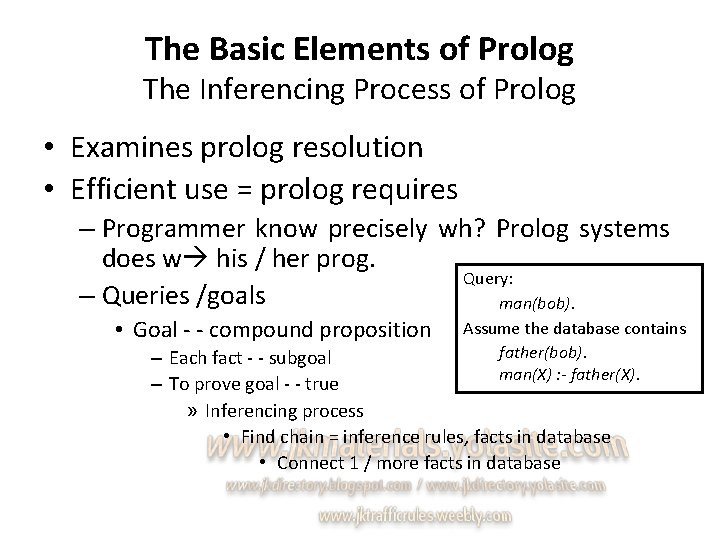The Basic Elements of Prolog The Inferencing Process of Prolog • Examines prolog resolution • Efficient use = prolog requires – Programmer know precisely wh? Prolog systems does w his / her prog. Query: – Queries /goals man(bob). • Goal - - compound proposition Assume the database contains father(bob). man(X) : - father(X). – Each fact - - subgoal – To prove goal - - true » Inferencing process • Find chain = inference rules, facts in database • Connect 1 / more facts in database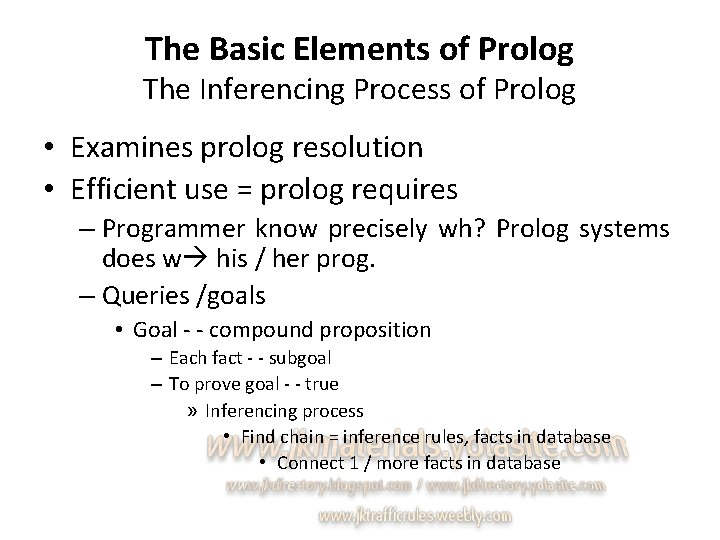The Basic Elements of Prolog The Inferencing Process of Prolog • Examines prolog resolution • Efficient use = prolog requires – Programmer know precisely wh? Prolog systems does w his / her prog. – Queries /goals • Goal - - compound proposition – Each fact - - subgoal – To prove goal - - true » Inferencing process • Find chain = inference rules, facts in database • Connect 1 / more facts in databaseThe Basic Elements of Prolog The Inferencing Process of Prolog • Examines prolog resolution • Efficient use = prolog requires – Programmer know precisely wh? Prolog systems does w his / her prog. – Queries /goals • Goal - - compound proposition – Each fact - - subgoal – To prove goal - - true » Inferencing process • Find chain = inference rules, facts in database • Connect 1 / more facts in database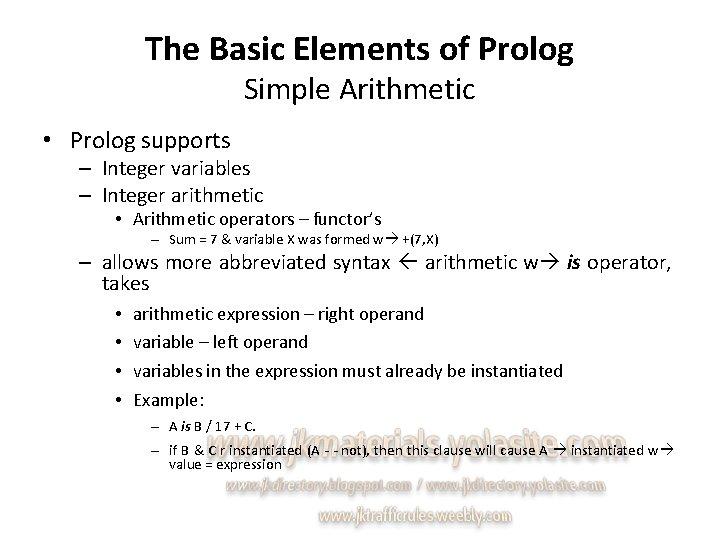The Basic Elements of Prolog Simple Arithmetic • Prolog supports – Integer variables – Integer arithmetic • Arithmetic operators – functor’s – Sum = 7 & variable X was formed w +(7, X) – allows more abbreviated syntax arithmetic w is operator, takes • • arithmetic expression – right operand variable – left operand variables in the expression must already be instantiated Example: – A is B / 17 + C. – if B & C r instantiated (A - - not), then this clause will cause A instantiated w value = expressionThe Basic Elements of Prolog Simple Arithmetic • Prolog supports – Integer variables – Integer arithmetic • Arithmetic operators – functor’s – Sum = 7 & variable X was formed w +(7, X) – allows more abbreviated syntax arithmetic w is operator, takes • • arithmetic expression – right operand variable – left operand variables in the expression must already be instantiated Example: – A is B / 17 + C. – if B & C r instantiated (A - - not), then this clause will cause A instantiated w value = expressionThe Basic Elements of Prolog Simple Arithmetic • How Prolog Produce Result? – Built-in structure – trace • Displays instantiations = values variables (each step) during attempt satisfy given goal • Used Understand & debug prolog prog. ’s – Consider tracing model understand trace • Describes prolog execution in terms = 4 events – call – beginning = an attempt satisfy goal (occurs) – exit – goal satisfied (occurs) – redo – backtrack causes attempt resatisfy goal (occurs) – fail – goal fails (occurs) trace. distance(chevy, Chevy_Distance). (1) 1 Call: distance(chevy, _0)? (2) 2 Call: speed(chevy, _5)? (2) 2 Exit: speed(chevy, 105) (3) 2 Call: time(chevy, _6)? (3) 2 Exit: time(chevy, 21) (4) 2 Call: _0 is 105*21? (4) 2 Exit: 2205 is 105*21 (1) 1 Exit: distance(chevy, 2205) Chevy_Distance = 2205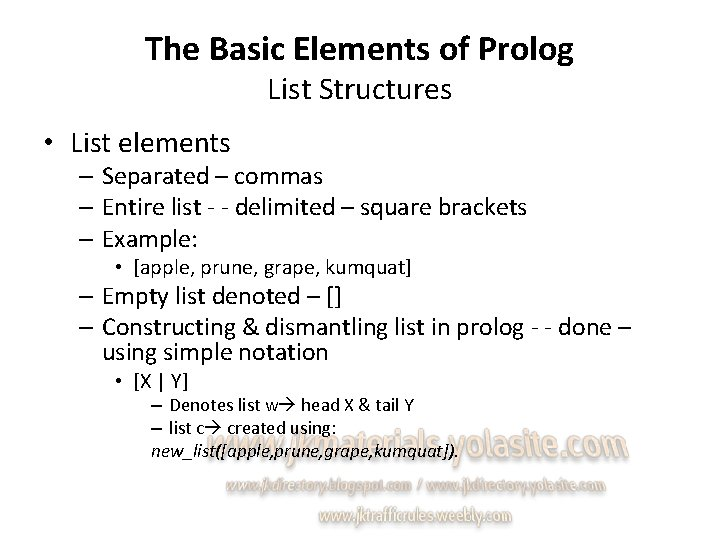The Basic Elements of Prolog List Structures • List elements – Separated – commas – Entire list - - delimited – square brackets – Example: • [apple, prune, grape, kumquat] – Empty list denoted – [] – Constructing & dismantling list in prolog - - done – using simple notation • [X | Y] – Denotes list w head X & tail Y – list c created using: new_list([apple, prune, grape, kumquat]).Functional Programming Languages Mathematical Functions • Mathematical function – Mapping = members = one set called domain set another set called range set – Function definition • Domain & range sets either explicitly | implicitly along w mapping – Mapping • Described – an expression | table • Mathematical Functions Characteristics: – evaluation order = mapping expressions • Controlled – recursion & conditional expressions ↔ sequencing & iterative repetition – Side effects & depend on any external values • Always map an element = domain same element = range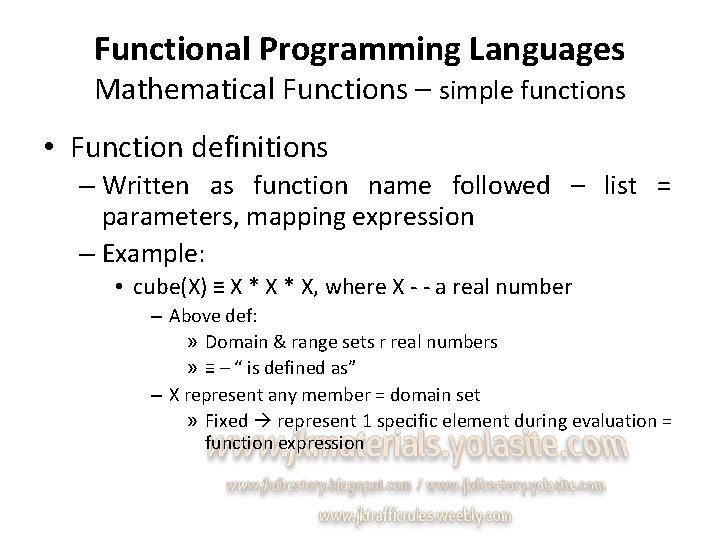Functional Programming Languages Mathematical Functions – simple functions • Function definitions – Written as function name followed – list = parameters, mapping expression – Example: • cube(X) ≡ X * X, where X - - a real number – Above def: » Domain & range sets r real numbers » ≡ – “ is defined as” – X represent any member = domain set » Fixed represent 1 specific element during evaluation = function expressionFunctional Programming Languages Mathematical Functions – simple functions • Function definitions – Written as function name followed – list = parameters, mapping expression – Example: • cube(X) ≡ X * X, where X - - a real number – Above def: » Domain & range sets r real numbers » ≡ – “ is defined as” – X represent any member = domain set » Fixed represent 1 specific element during evaluation = function expression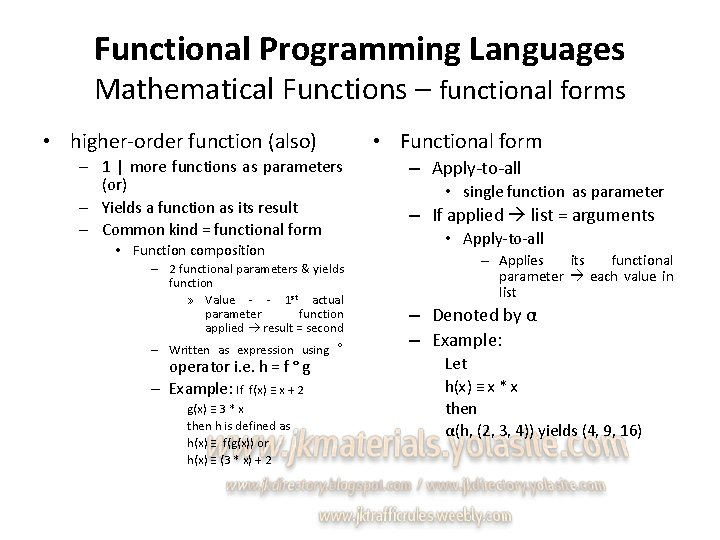Functional Programming Languages Mathematical Functions – functional forms • higher-order function (also) • Functional form – 1 | more functions as parameters (or) – Yields a function as its result – Common kind = functional form • Function composition – 2 functional parameters & yields function » Value - - 1 st actual parameter function applied result = second – Written as expression using operator i. e. h = f ° g – Example: If f(x) ≡ x + 2 g(x) ≡ 3 * x then h is defined as h(x) ≡ f(g(x)) or h(x) ≡ (3 * x) + 2 ° – Apply-to-all • single function as parameter – If applied list = arguments • Apply-to-all – Applies its functional parameter each value in list – Denoted by α – Example: Let h(x) ≡ x * x then α(h, (2, 3, 4)) yields (4, 9, 16)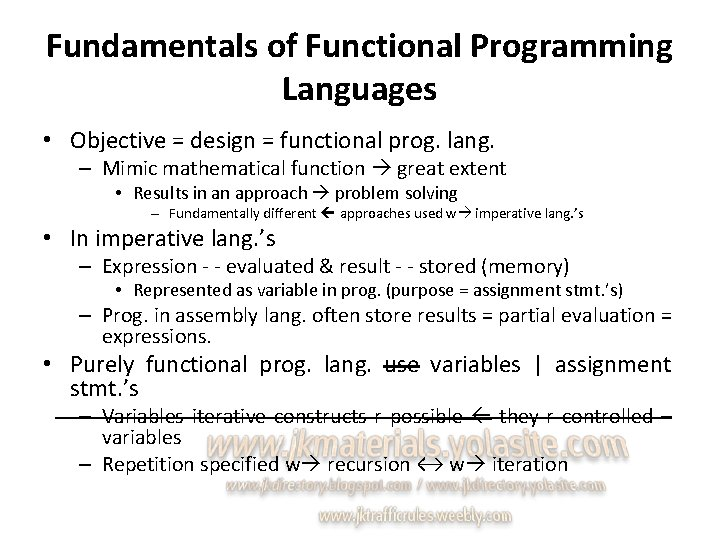Fundamentals of Functional Programming Languages • Objective = design = functional prog. lang. – Mimic mathematical function great extent • Results in an approach problem solving – Fundamentally different approaches used w imperative lang. ’s • In imperative lang. ’s – Expression - - evaluated & result - - stored (memory) • Represented as variable in prog. (purpose = assignment stmt. ’s) – Prog. in assembly lang. often store results = partial evaluation = expressions. • Purely functional prog. lang. use variables | assignment stmt. ’s – Variables iterative constructs r possible they r controlled – variables – Repetition specified w recursion ↔ w iterationLISP – Data Types and Structures • Oldest & most widely used – Developed – John Mc. Carthy at MIT (1959) • Data types & Structures: – Only 2 categories = data objects • Atoms & Lists (not types in sense that imperative lang. ’s have types) • List elements r pairs – 1 st part - - data = element(pointer an atom | a nested list) – 2 nd part c a pointer an atom | another element | empty list • LISP - - typeless lang. • Lists r specified – delimiting elements w () • Elements = simple list restricted atoms – (A B C D) • Nested list structures – (A (B C) D (E (F G))) • List - - referenced – pointer 1 st elementLISP – Data Types and Structures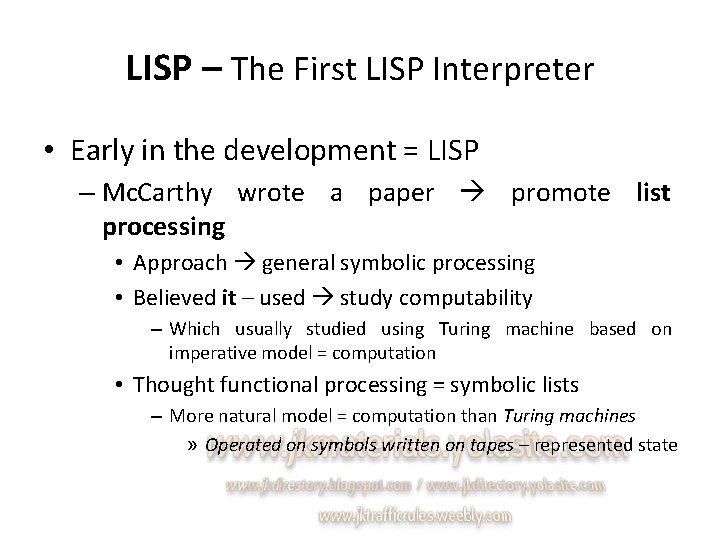LISP – The First LISP Interpreter • Early in the development = LISP – Mc. Carthy wrote a paper promote list processing • Approach general symbolic processing • Believed it – used study computability – Which usually studied using Turing machine based on imperative model = computation • Thought functional processing = symbolic lists – More natural model = computation than Turing machines » Operated on symbols written on tapes – represented stateLISP – The First LISP Interpreter • Early in the development = LISP – Mc. Carthy wrote a paper promote list processing • Approach general symbolic processing • Believed it – used study computability – Which usually studied using Turing machine based on imperative model = computation • Thought functional processing = symbolic lists – More natural model = computation than Turing machines » Operated on symbols written on tapes – represented state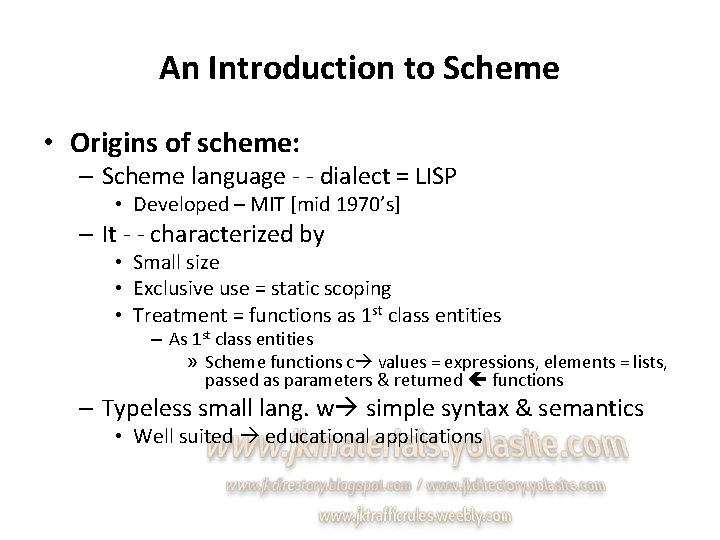An Introduction to Scheme • Origins of scheme: – Scheme language - - dialect = LISP • Developed – MIT [mid 1970’s] – It - - characterized by • Small size • Exclusive use = static scoping • Treatment = functions as 1 st class entities – As 1 st class entities » Scheme functions c values = expressions, elements = lists, passed as parameters & returned functions – Typeless small lang. w simple syntax & semantics • Well suited educational applicationsAn Introduction to Scheme • The Scheme Interpreter: – in interactive mode - - an infinite REPL – Repeatedly • Reads an expression • Interprets expression • Displays resulting value This form used in Ruby & Python – Expressions r interpreted • EVAL function – Literals evaluate themselves – Scheme prog. ’s stored in files c loaded & interpreted – Comments • Text following semicolon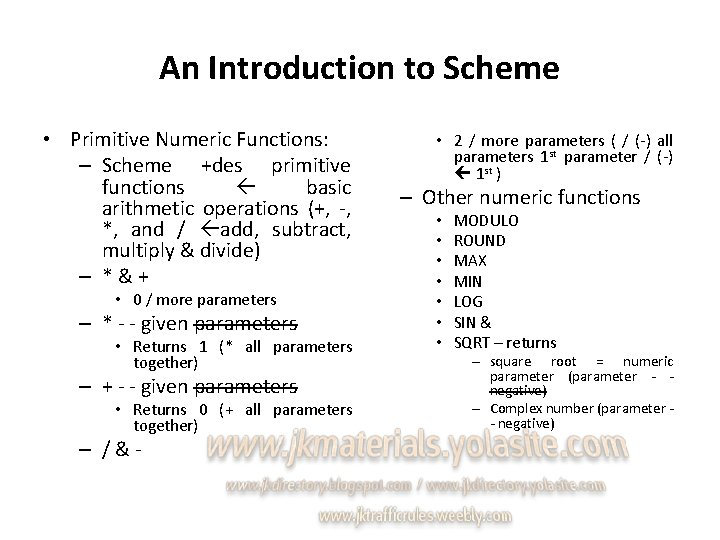An Introduction to Scheme • Primitive Numeric Functions: – Scheme +des primitive functions basic arithmetic operations (+, -, *, and / add, subtract, multiply & divide) – *&+ • 0 / more parameters – * - - given parameters • Returns 1 (* all parameters together) – + - - given parameters • Returns 0 (+ all parameters together) – /&- • 2 / more parameters ( / (-) all parameters 1 st parameter / (-) 1 st ) – Other numeric functions • • MODULO ROUND MAX MIN LOG SIN & SQRT – returns – square root = numeric parameter (parameter - negative) – Complex number (parameter - negative)An Introduction to Scheme • Scheme prog. - - collection = function definitions – H? define functions? • In scheme – Nameless function +des word LAMBDA (lambda expression) » Example: (LAMBDA (x) (* x x)) • Returns square root » Above function • Applied in same way – named functions – placing it in beginning = list • Contains actual parameters • Example: ((LAMBDA (x) (* x x))7) • bound variable – DEFINE » special form function – 2 basic needs • Bind name value • Bind name lambda expression » Example: (DEFINE pi 3. 14159)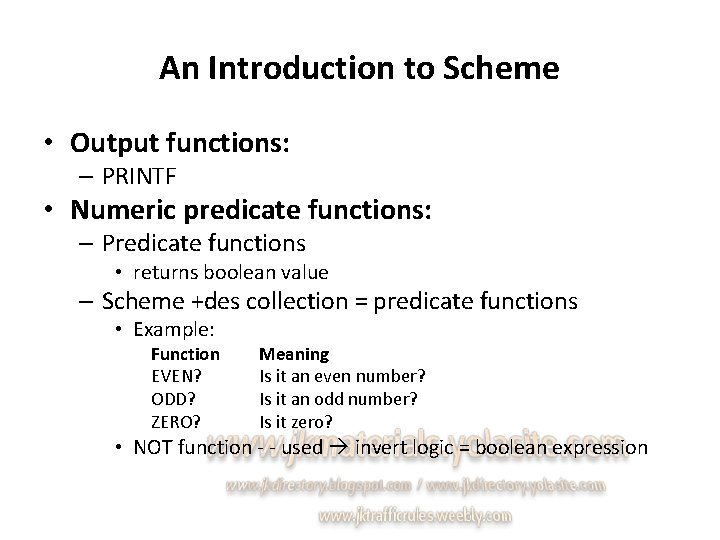An Introduction to Scheme • Output functions: – PRINTF • Numeric predicate functions: – Predicate functions • returns boolean value – Scheme +des collection = predicate functions • Example: Function EVEN? ODD? ZERO? Meaning Is it an even number? Is it an odd number? Is it zero? • NOT function - - used invert logic = boolean expression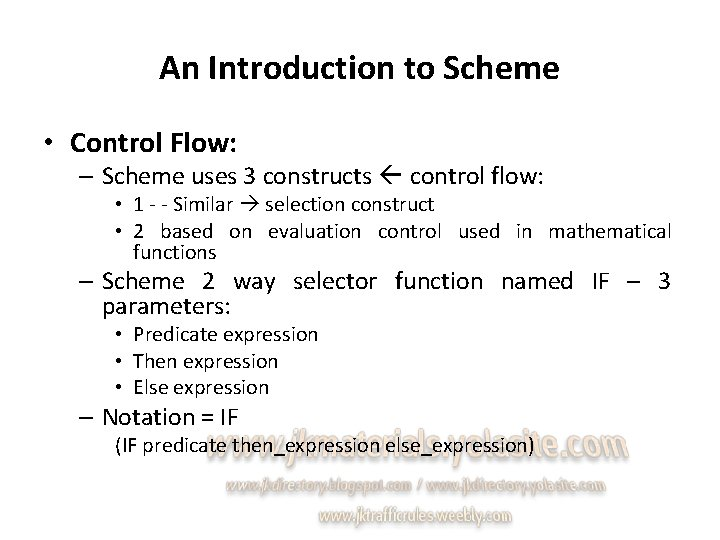An Introduction to Scheme • Control Flow: – Scheme uses 3 constructs control flow: • 1 - - Similar selection construct • 2 based on evaluation control used in mathematical functions – Scheme 2 way selector function named IF – 3 parameters: • Predicate expression • Then expression • Else expression – Notation = IF (IF predicate then_expression else_expression)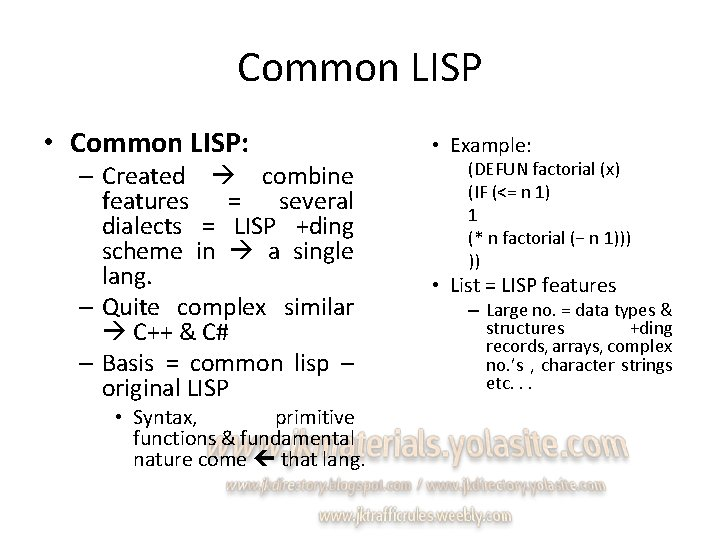Common LISP • Common LISP: – Created combine features = several dialects = LISP +ding scheme in a single lang. – Quite complex similar C++ & C# – Basis = common lisp – original LISP • Syntax, primitive functions & fundamental nature come that lang. • Example: (DEFUN factorial (x) (IF (<= n 1) 1 (* n factorial (− n 1))) )) • List = LISP features – Large no. = data types & structures +ding records, arrays, complex no. ’s , character strings etc. . .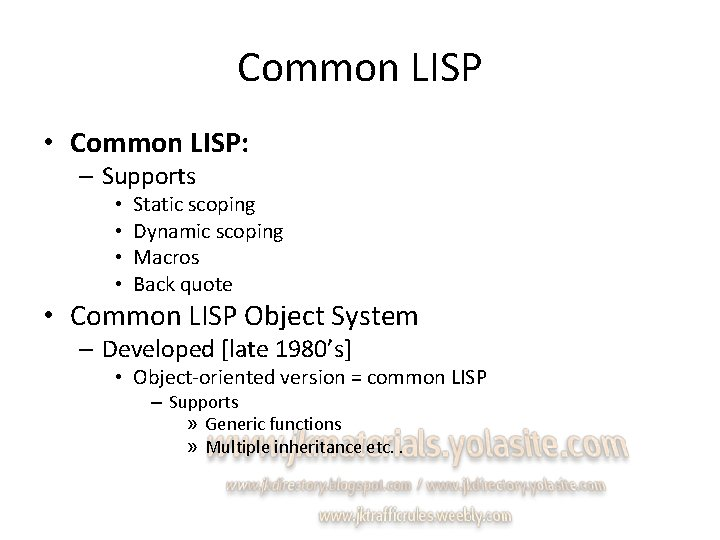Common LISP • Common LISP: – Supports • • Static scoping Dynamic scoping Macros Back quote • Common LISP Object System – Developed [late 1980’s] • Object-oriented version = common LISP – Supports » Generic functions » Multiple inheritance etc. .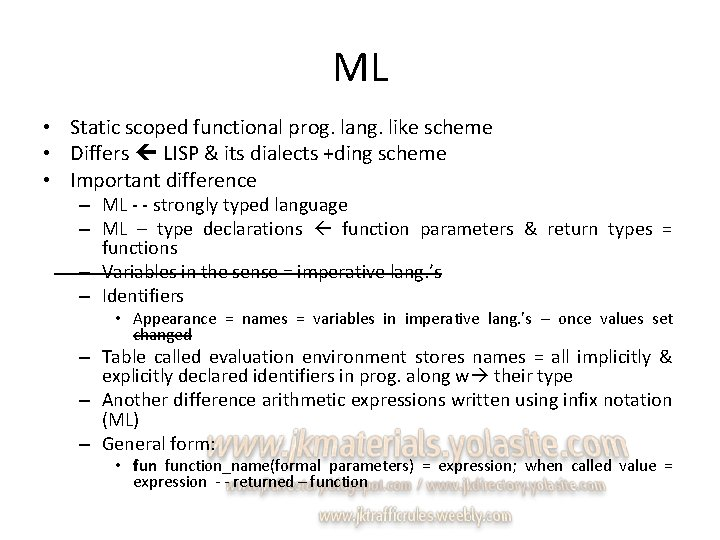ML • Static scoped functional prog. lang. like scheme • Differs LISP & its dialects +ding scheme • Important difference – ML - - strongly typed language – ML – type declarations function parameters & return types = functions – Variables in the sense = imperative lang. ’s – Identifiers • Appearance = names = variables in imperative lang. ’s – once values set changed – Table called evaluation environment stores names = all implicitly & explicitly declared identifiers in prog. along w their type – Another difference arithmetic expressions written using infix notation (ML) – General form: • function_name(formal parameters) = expression; when called value = expression - - returned – function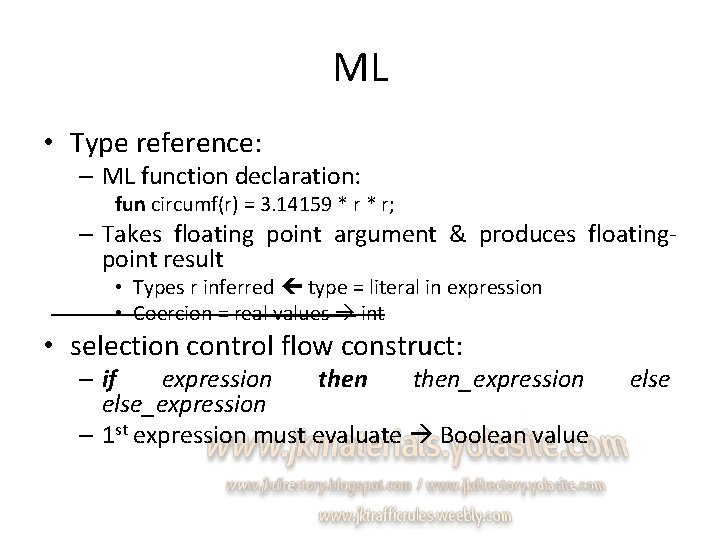ML • Type reference: – ML function declaration: fun circumf(r) = 3. 14159 * r; – Takes floating point argument & produces floatingpoint result • Types r inferred type = literal in expression • Coercion = real values int • selection control flow construct: – if expression then_expression else_expression – 1 st expression must evaluate Boolean value else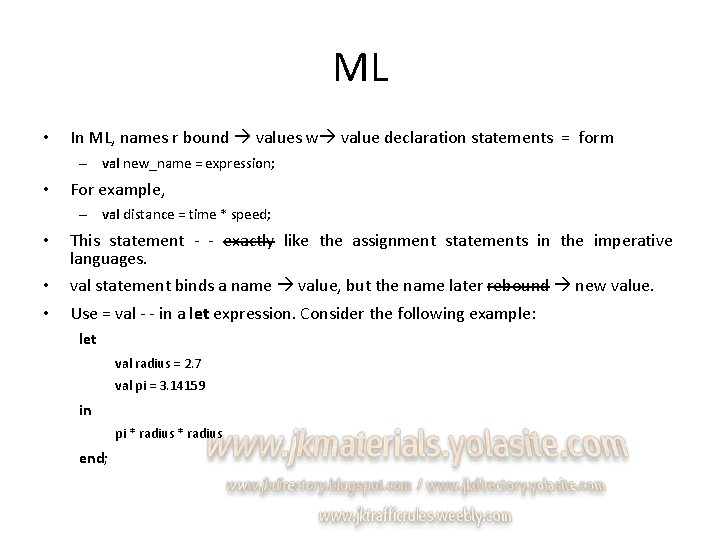ML • In ML, names r bound values w value declaration statements = form – val new_name = expression; • For example, – val distance = time * speed; • This statement - - exactly like the assignment statements in the imperative languages. • • val statement binds a name value, but the name later rebound new value. Use = val - - in a let expression. Consider the following example: let val radius = 2. 7 val pi = 3. 14159 in pi * radius end;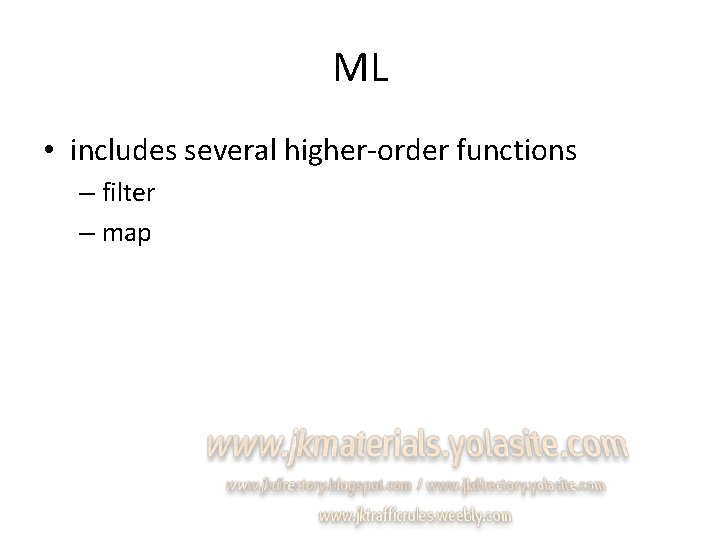ML • includes several higher-order functions – filter – map